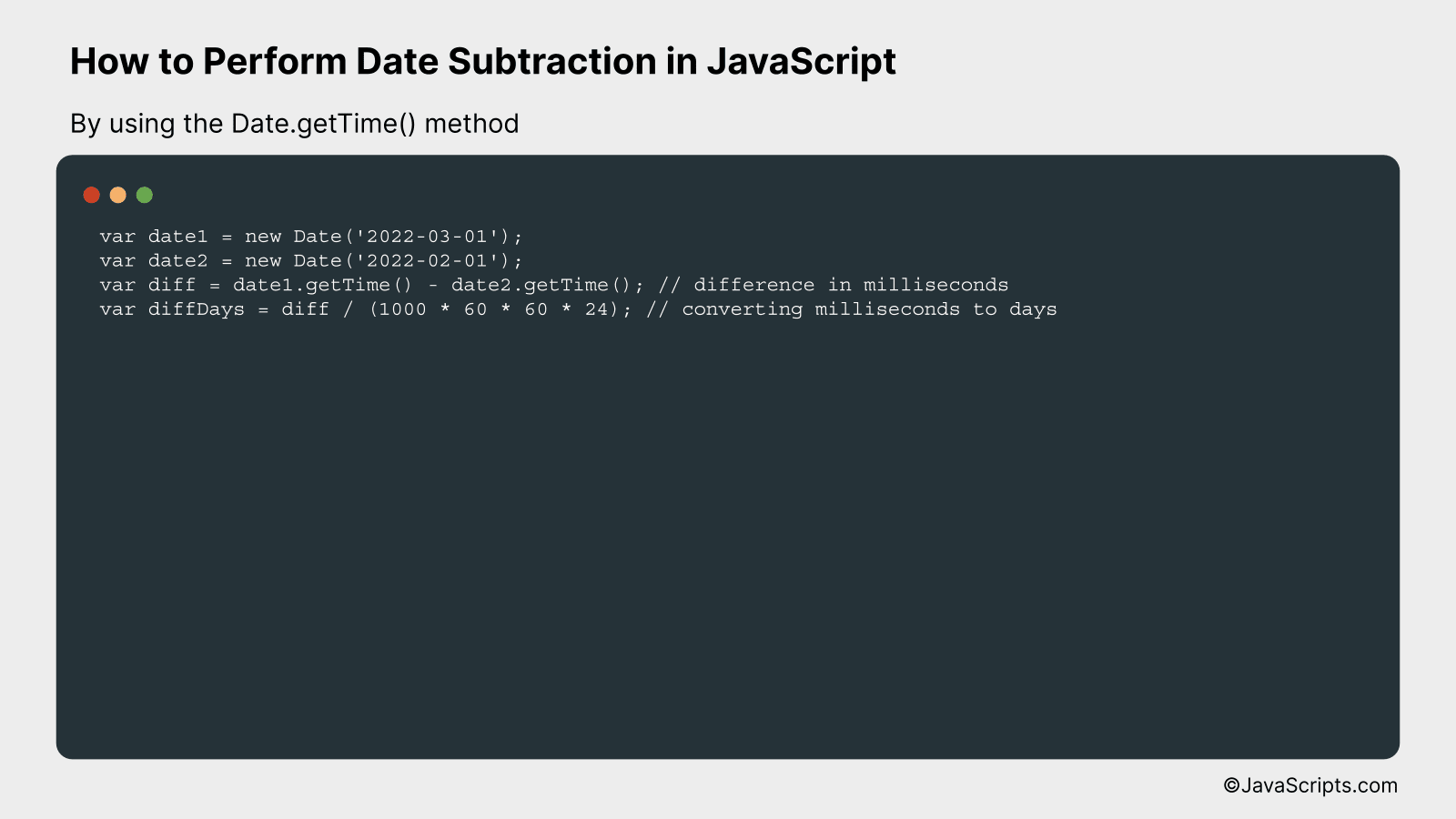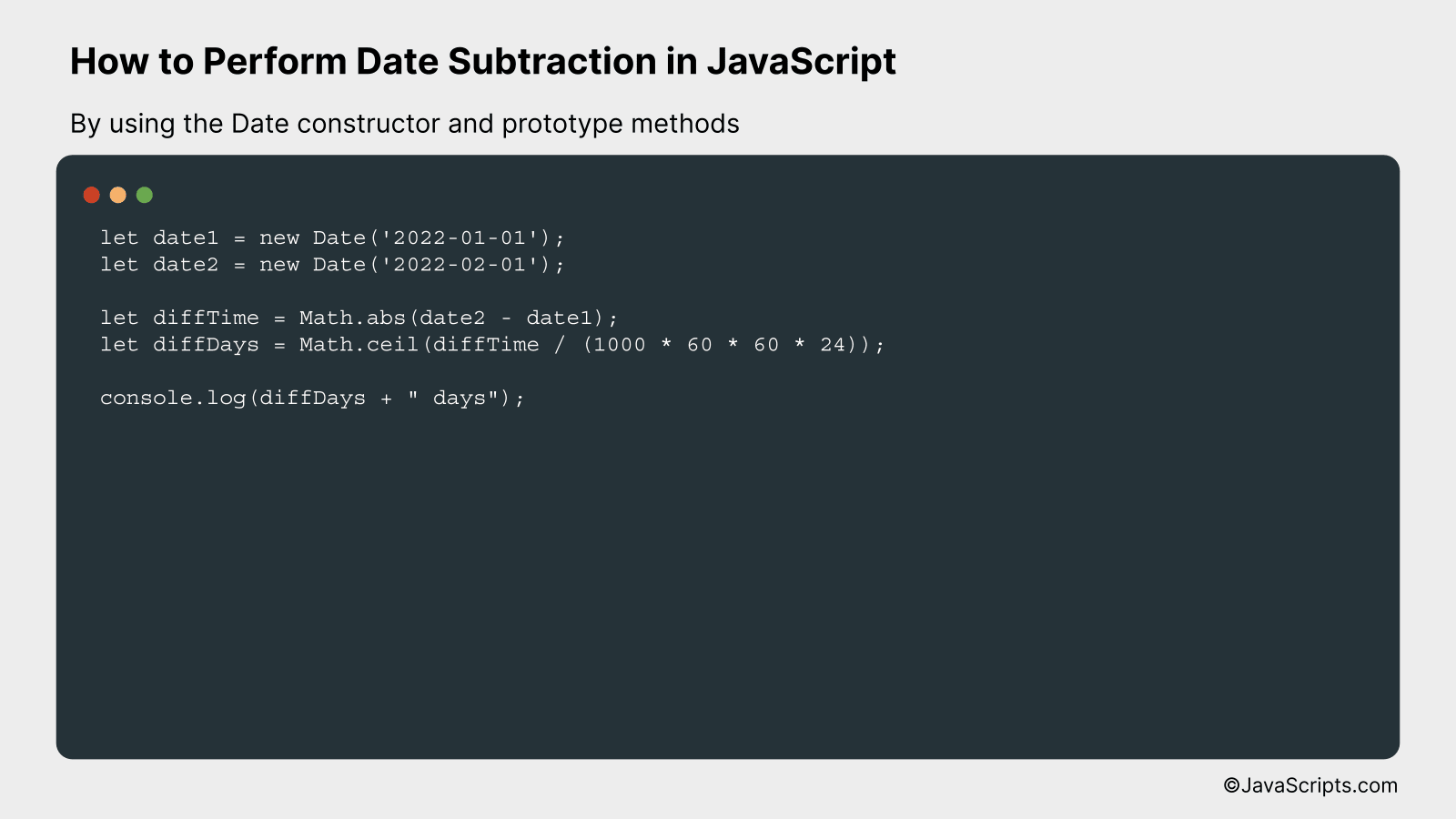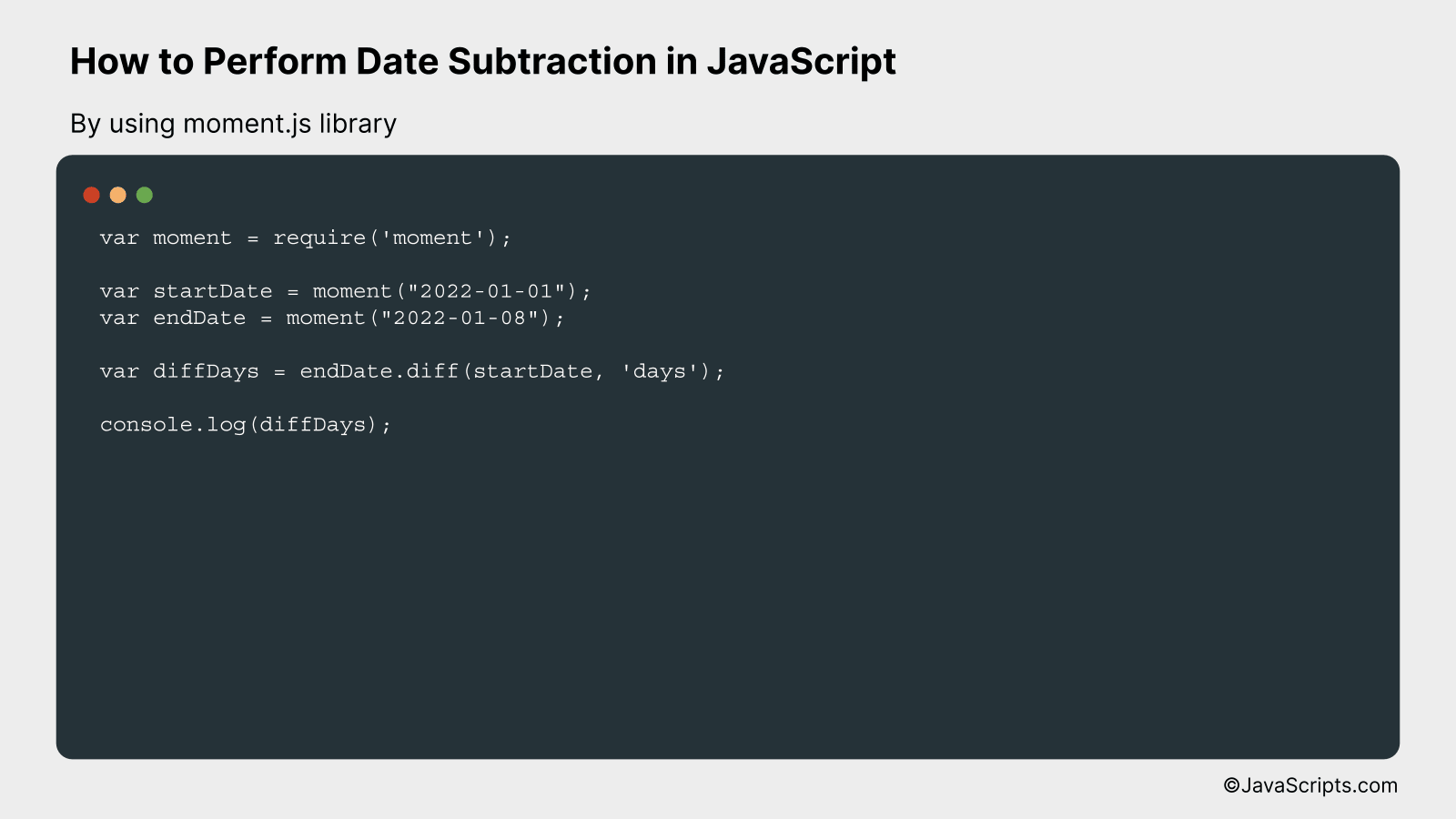# How to Perform Date Subtraction in JavaScript

JavaScript, the dynamic programming language, is famed for its versatility and functionality. It’s capable of handling complex tasks, be it manipulating web content, controlling multimedia, or managing dates and time, with grace and precision.

Are you trying to subtract dates in JavaScript but can’t quite crack the code? No worries, we’re here to help! We’ll break down this process step-by-step, using simple and easy-to-understand language, ensuring that by the end, you’ll be confidently subtracting dates in JavaScript.

In this post, we will be looking at the following 3 ways to perform date subtraction in JavaScript:

• By using the Date.getTime() method
• By using the Date constructor and prototype methods
• By using moment.js library

Let’s explore each…

## #1 – By using the Date.getTime() methodIn JavaScript, you can subtract two dates by using the Date.getTime() method, which returns the number of milliseconds since the Unix Epoch (January 1, 1970 00:00:00 UTC). The difference between two such returned values will give you the difference in milliseconds between the two dates.

``````
var date1 = new Date('2022-03-01');
var date2 = new Date('2022-02-01');
var diff = date1.getTime() - date2.getTime(); // difference in milliseconds
var diffDays = diff / (1000 * 60 * 60 * 24); // converting milliseconds to days
```
```

### How it works

The Date.getTime() method gives the time value in milliseconds that represents the date object. We subtract the getTime() value of the second date from the getTime() value of the first date. This gives us the difference in milliseconds. To convert this into days, we then divide the difference by the number of milliseconds in a day.

• The ‘new Date()’ constructor is used to create new date objects. The parameter passed to this constructor represents the specific date and time.
• The ‘getTime()’ method is called on these date objects to get their respective time values in milliseconds since the Unix Epoch.
• The ‘getTime()’ value of the second date is subtracted from the ‘getTime()’ value of the first date. This gives us the difference in milliseconds between the two dates.
• To convert the difference in milliseconds to days, we divide the difference by the number of milliseconds in one day (1000 milliseconds * 60 seconds * 60 minutes * 24 hours).

## #2 – By using the Date constructor and prototype methodsThe following process demonstrates how to compute the difference between two dates in JavaScript. It involves creating two Date objects, subtracting them, and then converting the result from milliseconds (default unit in JavaScript) to a more readable format. This concept becomes clear in the upcoming example.

``````
let date1 = new Date('2022-01-01');
let date2 = new Date('2022-02-01');

let diffTime = Math.abs(date2 - date1);
let diffDays = Math.ceil(diffTime / (1000 * 60 * 60 * 24));

console.log(diffDays + " days");
```
```

### How it works

The Date objects in JavaScript represent a single moment in time and are based on a time value that is the number of milliseconds since 1 January, 1970 UTC. When you subtract two dates, you get the difference in milliseconds. To convert this into a more understandable unit such as days, you need to divide the difference by the number of milliseconds in a day.

• Two date objects are created with different dates. `let date1 = new Date('2022-01-01');` and `let date2 = new Date('2022-02-01');` These dates are hardcoded for this example but can be any valid Date objects.
• The difference in time between these two dates is calculated with `let diffTime = Math.abs(date2 - date1);`. The `Math.abs()` function is used to get the absolute value of the difference, ensuring the result is always positive regardless of the dates’ order.
• The difference in days is then calculated by dividing the difference in time (in milliseconds) by the number of milliseconds in a day with `let diffDays = Math.ceil(diffTime / (1000 * 60 * 60 * 24));`. The `Math.ceil()` function is used to round up to the nearest whole number, as a part of a day is generally considered a full day in these calculations.
• Finally, the difference in days is printed to the console with `console.log(diffDays + " days");`

## #3 – By using moment.js libraryIn JavaScript, using the moment.js library, you can subtract dates by using the subtract method, which takes two arguments: the number of units to subtract and the type of unit. For example, to subtract 7 days from the current date, we use moment().subtract(7, ‘days’).

``````
var moment = require('moment');

var startDate = moment("2022-01-01");
var endDate = moment("2022-01-08");

var diffDays = endDate.diff(startDate, 'days');

console.log(diffDays);
```
```

### How it works

The diff method from the Moment.js library is used to calculate the difference between two dates. The first argument to the method is the earlier date, and the second argument is the unit of time to calculate the difference in (such as ‘days’, ‘months’, ‘years’, etc.).

• First, the Moment.js library is imported using require(‘moment’).
• Then, two dates are defined using the moment function with the desired date in the format “YYYY-MM-DD”. In this case, startDate is set to “2022-01-01” and endDate is set to “2022-01-08”.
• The diff method is then called on the endDate object, with startDate as the first argument and ‘days’ as the second argument, which tells the method to calculate the difference in days.
• The result is stored in the diffDays variable.
• Finally, the difference in days between the startDate and endDate is printed to the console using console.log(diffDays).

Related:

In sum, date subtraction in JavaScript isn’t as complex as it initially seems. By utilizing in-built functions like Date(), we can manipulate dates quite easily. It’s all about understanding the milliseconds conversion and taking advantage of the system’s built-in methods.

With practice, you’ll find date subtraction becoming second nature. So, whenever working with dates in JavaScript, remember these simple steps. Keep experimenting with different scenarios, and you’ll become adept at it in no time.